# SSAT Elementary Level Math : How to find the whole from the part

## Example Questions

### Example Question #3 : Identifying Numbers

What number is two hundred?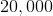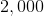Explanation:in word form is two hundred.

### Example Question #4 : Identifying Numbers

What number is nine hundred?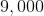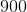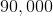Explanation:in word form is nine hundred.

### Example Question #5 : Identifying Numbers

What number is forty-three?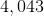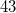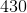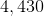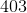Explanation:in word form is forty-three.

### Example Question #6 : Identifying Numbers

What number is eight hundred four?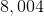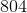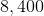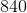Explanation:in word form is eight hundred forty-four.

### Example Question #1 : Compare Two Three Digit Numbers: Ccss.Math.Content.2.Nbt.A.4

Which statement is true?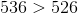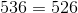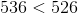There is no true statementExplanation:

The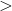sign means greater than.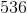is greater than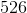because of the number in the tens place.has a greater value than, which makes 536 have a greater value than### Example Question #1 : Compare Two Three Digit Numbers: Ccss.Math.Content.2.Nbt.A.4

Which statement is true?

There is no true statement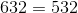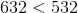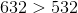Explanation:

Thesign means greater than.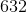is greater than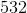because of the number in the hundreds place.has a greater value than, which makesgreater than### Example Question #3 : Compare Two Three Digit Numbers: Ccss.Math.Content.2.Nbt.A.4

Which statement is true?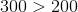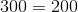There is no true statement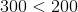Explanation:

Thesign means greater than.is greater thanbecause of the number in the hundreds place.has a greater value than, which makesgreat than### Example Question #4 : Compare Two Three Digit Numbers: Ccss.Math.Content.2.Nbt.A.4

Which statement is true?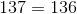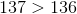There is no true statement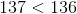Explanation:

Thesign means greater than.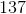is greater than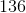because of the number in the ones place.has a greater value than, which makesgreater than### Example Question #1 : Compare Two Three Digit Numbers: Ccss.Math.Content.2.Nbt.A.4

Which statement is true?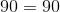There is no true statement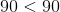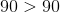Explanation:

Thesign means equal to. Becauseandare the same number they are equal to each other. They can’t be less than or greater than each other because their value is the same.

### Example Question #6 : Compare Two Three Digit Numbers: Ccss.Math.Content.2.Nbt.A.4

Which statement is true?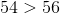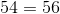There is no true statement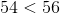The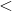sign means less than.is less thanbecause of the number in the ones place.has a lesser value than, which makesless than